# 12.2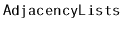: A Graph as a Collection of Lists

Adjacency list representations of graphs take a more vertex-centric approach. There are many possible implementations of adjacency lists. In this section, we present a simple one. At the end of the section, we discuss different possibilities. In an adjacency list representation, the graph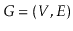is represented as an array,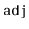, of lists. The list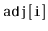contains a list of all the vertices adjacent to vertex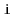. That is, it contains every index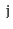such that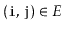.

  int n;

(An example is shown in Figure 12.3.) In this particular implementation, we represent each list inas a subclass of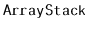, because we would like constant time access by position. Other options are also possible. Specifically, we could have implementedas a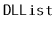.

Figure 12.3: A graph and its adjacency lists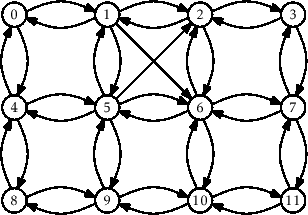0 1 2 3 4 5 6 7 8 9 10 11 1 0 1 2 0 1 5 6 4 8 9 10 4 2 3 7 5 2 2 3 9 5 6 7 6 6 8 6 7 11 10 11 5 9 10 4

The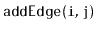operation just appends the valueto the list:

  void addEdge(int i, int j) {
}

This takes constant time.

The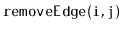operation searches through the listuntil it findsand then removes it:

  void removeEdge(int i, int j) {
for (int k = 0; k < adj[i].size(); k++) {
if (adj[i].get(k) == j) {
return;
}
}
}

This takes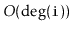time, where(the degree of) counts the number of edges in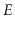that haveas their source.

The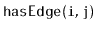operation is similar; it searches through the listuntil it finds(and returns true), or reaches the end of the list (and returns false):

  bool hasEdge(int i, int j) {
}

This also takestime.

The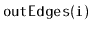operation is very simple; it copies the values ininto the output list:

  void outEdges(int i, LisT &edges) {
for (int k = 0; k < adj[i].size(); k++)
}

This clearly takestime.

The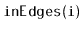operation is much more work. It scans over every vertexchecking if the edgeexists and, if so, addingto the output list:

  void inEdges(int i, LisT &edges) {
for (int j = 0; j < n; j++)
}

This operation is very slow. It scans the adjacency list of every vertex, so it takestime.

The following theorem summarizes the performance of the above data structure:

Theorem 12..2   Thedata structure implements the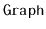interface. Ansupports the operations
•in constant time per operation;
•andintime per operation;
•intime per operation; and
•in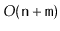time per operation.
The space used by ais.

As alluded to earlier, there are many different choices to be made when implementing a graph as an adjacency list. Some questions that come up include:

• What type of collection should be used to store each element of? One could use an array-based list, a linked-list, or even a hashtable.
• Should there be a second adjacency list,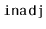, that stores, for each, the list of vertices,, such that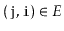? This can greatly reduce the running-time of theoperation, but requires slightly more work when adding or removing edges.
• Should the entry for the edge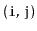inbe linked by a reference to the corresponding entry in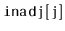?
• Should edges be first-class objects with their own associated data? In this way,would contain lists of edges rather than lists of vertices (integers).
Most of these questions come down to a tradeoff between complexity (and space) of implementation and performance features of the implementation.

opendatastructures.org